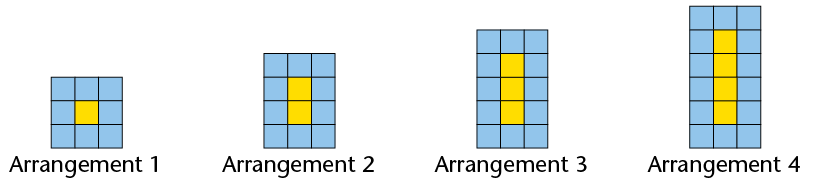• Blue and yellow square tiles are combined to form the above arrangements.

1. How many yellow tiles are there in each arrangement?

2. How many blue tiles are there in each arrangement?

3. If more arrangements are made in the same way, how many blue tiles and how many yellow tiles will there be in arrangement 5? Check your answer by drawing the arrangement on the grid on the right.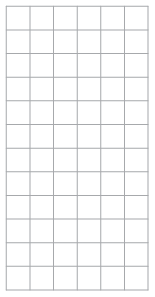4. Complete this table.

 Number of yellow tiles 1 2 3 4 5 8 Number of blue tiles
5. How many blue tiles will there be in a similar arrangement with 26 yellow tiles?

6. How many blue tiles will there be in a similar arrangement with 100 yellow tiles?

1. In these arrangements there are red tiles too. Complete this table.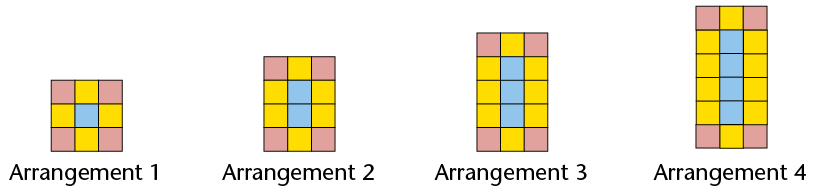Number of blue tiles 1 2 3 4 5 6 7 Number of yellow tiles Number of red tiles
2. How many red tiles are there in each arrangement?

3. How many yellow tiles are there in each arrangement?

The number of red tiles in arrangements like those in question 2 is constant. It is always 4, no matter how many blue and yellow tiles there are. The number of blue tiles is different for different arrangements. We can say the number of blue tiles varies. We can also say the number of blue tiles is a variable.

• Is the number of yellow tiles in the above arrangements a constant or is it a variable?

• Look at these three arrangements. They consist of black squares, grey squares and white squares.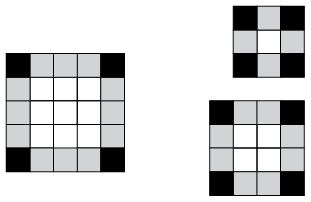1. Draw another arrangement of the same kind, but with a different length, on the grid provided on the right.

2. Describe what is constant in these arrangements.

3. What are the variables in these arrangements?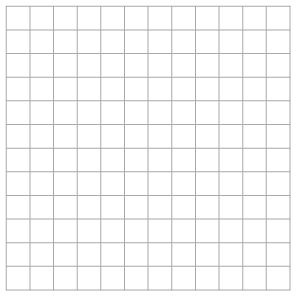The smallest arrangement above may be called arrangement 1, the next bigger one may be called arrangement 2, and so on.

1. Complete the table for arrangements like those in question 4.

 Arrangement number 1 2 3 4 5 6 7 10 20 Number of black squares Number of grey squares Number of white squares
2. How many grey squares do you think there will be in arrangement 15? Explain your answer.

3. How many black squares do you think there will be in arrangement 15? Explain your answer.

4. How many white squares do you think there will be in arrangement 15? Explain your answer.

• What are the next five numbers in each of the above patterns?

1. Draw the next arrangement that follows the same pattern.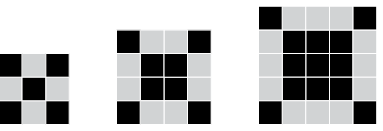2. How many black tiles are there in the arrangement you have drawn?

3. How many black tiles will there be in each of the next four arrangements?

• Number of grey squares

20

36

52

Number of white squares

256

225

625

1. Make two more arrangements of black and grey squares so that a pattern is formed.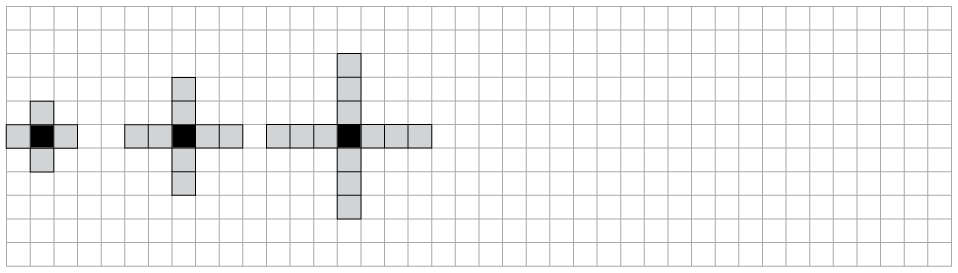2. Is there a constant in your pattern? If yes, what is its value?

3. Is there a variable in your pattern? If yes, give the values of the variable.

1. Make three more arrangements with dots to form the sequence 1; 3; 6; 10; 15 . . .2. How many dots will there be in the sixth and seventh arrangements? Explain how you got your answer.

3. How many dots are there in arrangements 1 and 2 together?

4. How many dots are there in arrangements 2 and 3 together?

5. How many dots are there in arrangements 3 and 4 together?

6. How many dots are there in arrangements 4 and 5 together?

7. Describe the pattern in your answers for (c), (d), (e) and (f).

1. Draw two more arrangements to make a pattern.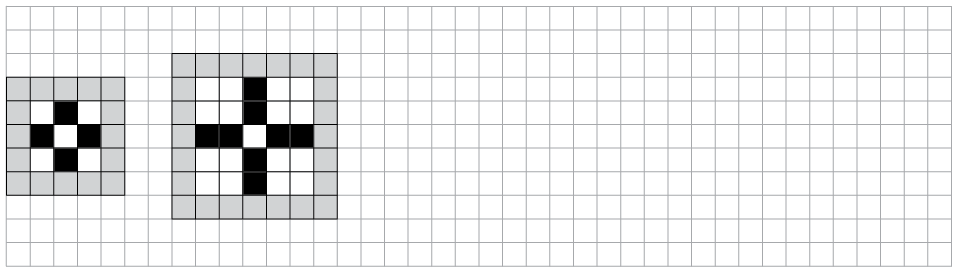2. What are the variables in your pattern?

3. The number of black squares is a variable in these arrangements. The value of this variable is 4 in the first arrangement and 8 in the second arrangement. What is the value of this variable in the third arrangement?

4. What are the values of each of the variables in the fifth arrangement in your pattern? Explain your answers.

1. Now make a pattern of your own.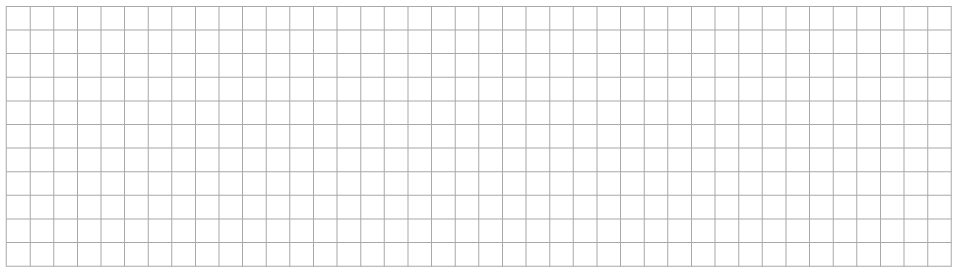2. Use this table to describe the variables in your pattern, and their values.

 Arrangement number 1 2 3 4 5 6
1. Write the next three numbers in each of the sequences below.

Sequence A: 5 9 13 17 21

Sequence B: 5 10 20 40 80

Sequence C: 5 10 17 26 37

2. Describe the differences in the ways in which the three sequences are formed.

• You will now make a sequence with the first term 5. Write 5 on the left on the line below. Then add 8 to the first term (5) to form the second term of your sequence. Write the second term next to the first term (5) in the line below. Now add 8 to the second term to form the third term. Continue like this to form ten more terms.

The numbers in a sequence are also called the terms of the sequence.

A sequence can be formed by repeatedly adding or subtracting the same number. In this case the difference between consecutive terms in a sequence is constant. A sequence can be formed by repeatedly multiplying or dividing. In this case the ratio between consecutive terms is constant. A sequence can also be formed in such a way that neither the difference nor the ratio between consecutive terms is constant.

To write more terms of sequence A in question 1(a), you added 4 repeatedly.

To write more terms of sequence B in question 1(a), you multiplied by 2 repeatedly.

To write more terms of sequence C in question 1(a) you did not add the same number each time, nor did you multiply by the same number.

• Write the next three terms of each sequence. In each case also describe what the pattern is, for example "there is a constant difference of -5 between consecutive terms".

1. 100; 92; 84; 76;

2. 1; 4; 9; 16;

3. 2; 8; 18; 32;

4. 3; 6; 11; 18;

5. 640; 320; 160;

6. 1; 2; 4; 7; 11;

• In each case, follow the instruction to make a sequence with eight terms.

• Term number

1

2

3

4

5

6

7

8

9

10

Term value

5

13

21

29

37

45

53

61

69

77

• In each case make a sequence by following the instructions. Write the term numbers and the term values in the given table.

1. Term 1 = 10. Add 15 repeatedly.

 Term number Term value
2. Term 1 = 10. Term value = 15 $\times$ term number - 5.

 Term number Term value
3. Term 1 = 10. Multiply by 2 repeatedly.

 Term number Term value
4. Term 1 = 20. $\text{Term value} = 10 \times 2^\text{term number}$

 Term number Term value
5. Term 1 = 10. Term value = $10 \times 2^{\text{term number}-1}$

 Term number Term value
6. Term 4 = 30. Add 5 repeatedly.

 Term number Term value
• Instructions for forming a sequence are given in two different ways in question 5. How would you describe the two different ways for giving instructions to form a sequence?

• A description of the relationship between consecutive terms. In other words the calculations that you do to a term to produce the next term, as in questions 5(a), (c) and (f) on the previous page. The first (or another) term must be given. This kind of formula has two parts, the first term, and the relationship between terms.
• A description of the relationship between the value of the term and its position in the sequence. This relationship describes the calculations that can be done on the term number to produce the term value, as in question 5(b), (d) and (e) on the previous page.
• Choose any whole number smaller than 10 as the first term of a sequence.

1. Use your chosen first term to form a sequence by adding 5 repeatedly.

2. Multiply each term number below by 5 to form a sequence:

 Term number 1 2 3 4 5 6 7 8 Term value
3. What is similar about the two sequences you have formed?

4. Now fill in your own sequence in the same table:

 Term number 1 2 3 4 5 6 7 8 Term value in (b) Term value of your own sequence in (a)
5. What must you add to or subtract from each term value in (b) to get the same sequence as the one you made in (a)?

6. Fill in the following to write a formula for each sequence:

For the sequence in (b): Term value =______ (term number) ______

For the sequence in (a): Term value =______ (term number) ______

• Now you are going to repeat what you did in question 1, with a different set of sequences.

In this sequence, the term number is multiplied by 3 to get the term value.

 Term number 1 2 3 4 5 6 7 8 Term value 3 6 9 12 15 18 21 24

Now make a formula describing the relationship of the term value to the term number for each of these sequences:

1. The sequence that starts with 8 and is formed by adding 3 repeatedly.

2. The sequence that starts with 12 and is formed by adding 3 repeatedly.

3. The sequence that starts with 2 and is formed by adding 3 repeatedly.

• Write the first eight terms of each of the following sequences, and in each case describe how each term can be calculated from the previous term.

1. $\text{Term value} = 10 \times \text{term number} + 5$

 Term number 1 2 3 4 5 6 7 8 Term value

2. $\text{Term value} = 5 \times \text{term number} - 3$

 Term number 1 2 3 4 5 6 7 8 Term value

• For each sequence, write a formula to obtain each term from the previous term, and also try to write formula which relates each term to its position in the sequence. Check both your formulae by applying them, and write the results in the table.

1. 7; 11; 15; 19; 23; 27; 31; 35; 39; 43

A. Relationship between consecutive terms:

B. Relationship between term value and its position in sequence:

 Term number 1 2 3 4 5 Term value using A Term value using B
2. 60; 57; 54; 51; 48; 45; 42; 39; 36

A. Relationship between consecutive terms:

B. Relationship between term value and its position in sequence:

 Term number 1 2 3 4 5 Term value using A Term value using B
3. 1; 2; 4; 8; 16; 32; 64; 128

A. Relationship between consecutive terms:

B. Relationship between term value and its position in sequence:

 Term number 1 2 3 4 5 Term value using A Term value using B